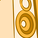# QlikView App Dev

Discussion Board for collaboration related to QlikView App Development.

Announcements
Become an analytics expert with Qlik's new 15 week course: Applied Data Analytics using Qlik Sense. READ MORE
cancel
Showing results for
Did you mean:Contributor II

## Aggregation function Problem

Hello Qview Community!

I'm relatively new to qlikvew and I did a couple of tutorials, but now I've reached a problem that I can't solve.

I have a big DB and there is an expression I can't seem to do properly. This is a simplified part of it:

P&LYrMthValue

Logistics

2014011000
Commission201401500
Labor201401300
Sales2014018000
Other2014013000
Logistics2014021200
Comission201402

400

Labor201402400
Sales2014029000
Other2014021100

What I want to do is a Pivot table where the dimensions are [P&L] and [Yr/Mth]. In the expressions I'd like to see sum(Value) and it's ratio to the sales relative to that month.

I've tried the following expression: sum(Value) / sum({\$<[P&L] = {'Sales'}>} Value). But I only get information in the "Sales" line.

I see that this may be obvious to some, but I still don't have the Qlikview "mentality" going on.

I also tried aggr(sum( {\$< [P&L]={'Sales'} >} Value), [P&L], [Yr/Mth]). I tried using TOTAL but it gives me the total for all the months and I'd like it to calculate for each month, without having to select that specific month

Thank you very much!

1 Solution

Accepted SolutionsEmployee

Here is a solution for the % of total by YrMnth

sum( Value)

/

sum( total <YrMth> {\$<[P&L]={'Sales'}>} Value)4 RepliesEmployee

Here is a solution for the % of total by YrMnth

sum( Value)

/

sum( total <YrMth> {\$<[P&L]={'Sales'}>} Value)Contributor II
Author

Thank you so much for the fast response!Contributor II
Author

Hello, I have another question now!

I tried making a bar chart out of this information now and it doesnt respect my selections.The excluded selections in my dimensions still appear in my graph.

How can I solve this?Employee

So a line chart gets tricky with 2 dimensions and 2 measures. (which is what we have above). 2 dimensions and 1 measure is ok and 1 dimension and 2 measures.  You can do 2 dims and 2 measures in a stacked bar chart where both measures are on the same axis... but that is pretty specific.

Do you have a picture of the line chart you have in mind ?  I'm thinking maybe have 2 charts...1 for each expression.